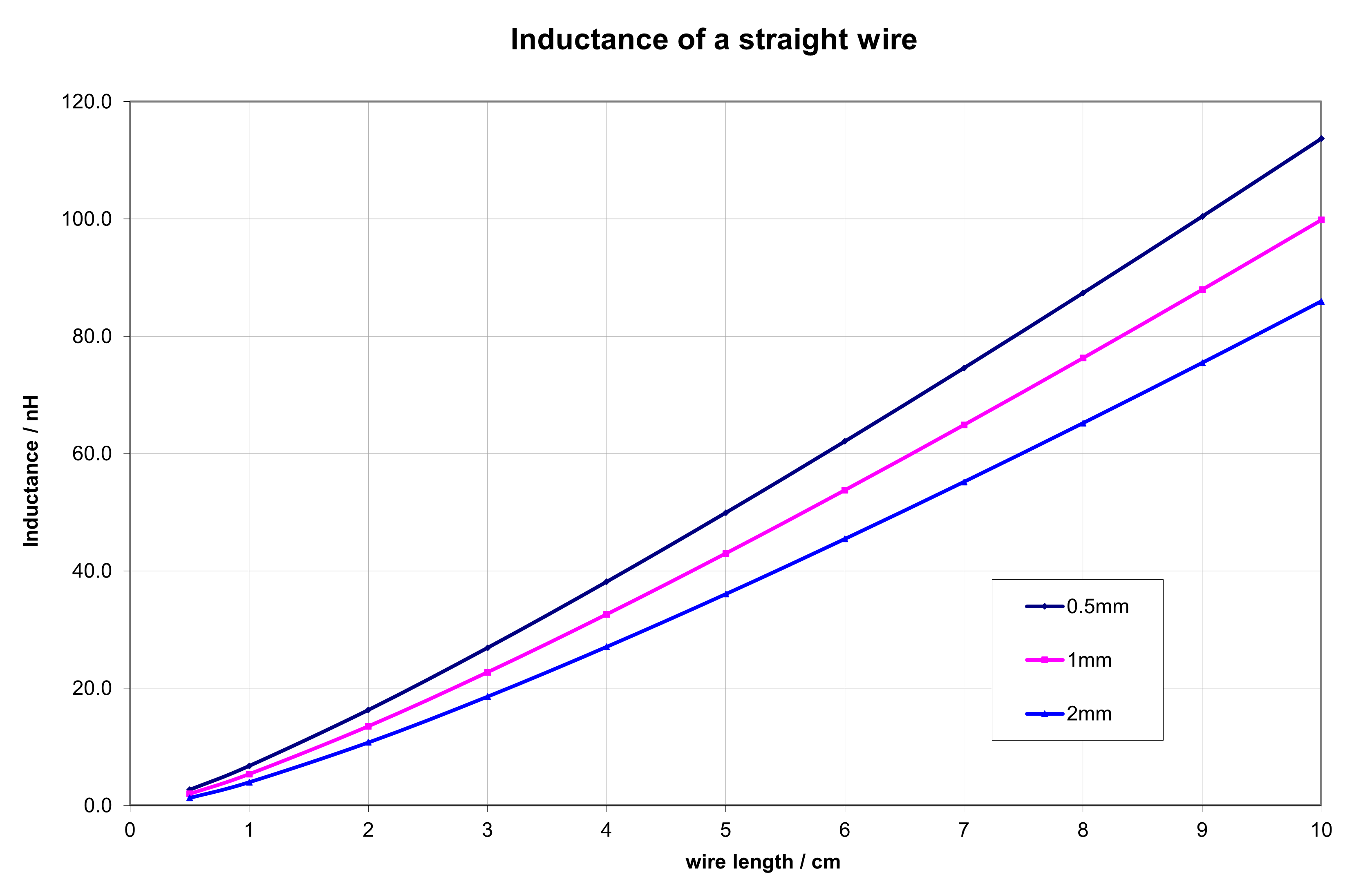### Inductance of a straight wire

This project was originally made in 2011 in Excel format. I converted it to html and Java script in 2021.

When wiring RF-circuits, connecting straight wires act like additional inductors. Following calculator can help to estimate the magnitude of those stray inductances, to be taken into account.

#### Formulas

Lac = 0.2*L*[ln(4*L/d)-1.00] nH , where Lac is the inductance in nH, L is the length of the wire and d the diameter of the wire, both in mm.

#### Calculator

Give length L of the wire in mm.

❶.

Give diameter d of the wire in mm

❷.

Inductance Lac is:References:

Pekka Ketonen 17.5.2021